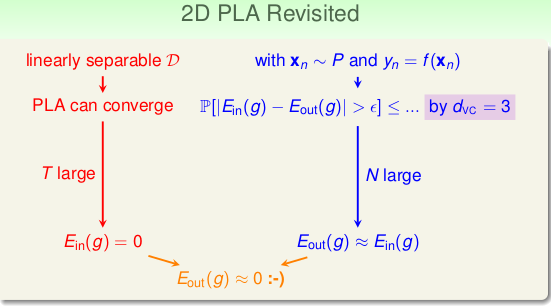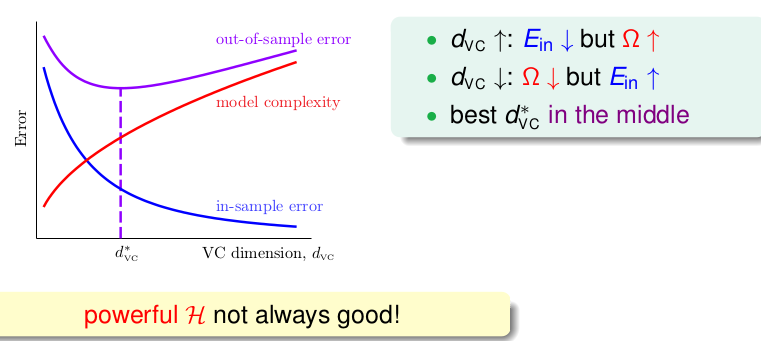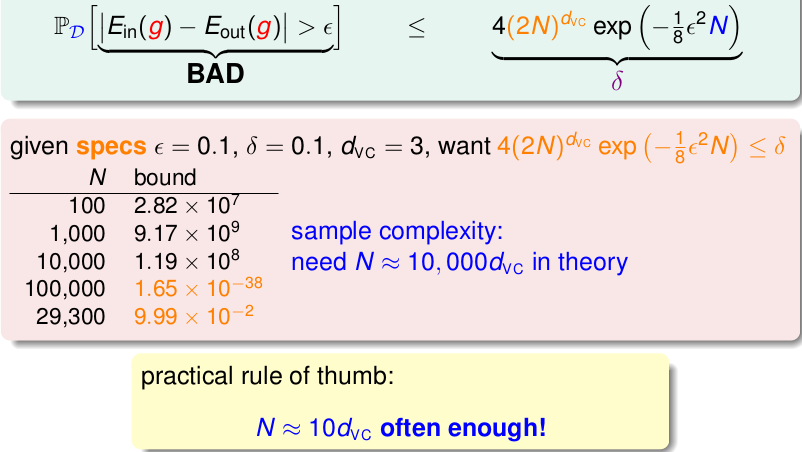# 机器学习基石笔记(3)

2016.11.30更新: VC维

## 最大的最大:B(N,K)

• $N < K$: every $m_{}{N} = 2^N$
• $N \geq K$: every $m_{\mathcal{H}}(N) < 2^N$，对于 N > K的D, 显然$m < 2^N$

## VC Dimension

\begin{align*} \mathbb{P}[BAD] &= \mathbb{P}[\exists h \in \mathcal{H}\text{ s.t. } |E_{in}(h)-E_{out}(h)|\gt \epsilon] \\ &\leq 4m_{\mathcal{H}}(2N)exp(-\frac{1}{8}\epsilon^2N) \\ &= 4(2N)^{d_{vc}} exp(-\frac{1}{8}\epsilon^2N)\end{align*} 那么怎么样才可以说明机器学到了东西呢？有以下三点:

• 存在break point即 $d_{vc}(\mathcal{H})$是有限的，这样的$\mathcal{H}$才是good的
• 训练数据足够多: N 够大，这样vc bound 可以约束$E_{in} = E_{out}$
• 算法$\mathcal{}A$ 可以找到一个$E_{in}$最小的hpic

$\epsilon= \sqrt{\frac{8}{N}ln(\frac{4(2N)^{d_{vc}}}{\delta})}$它就是$E_{in}$$E_{out}$的差距，对于好数据来说，有很大的几率：$\| E_{in} - E_{out} \| < \epsilon$，代入 $\epsilon$即:

$E_{in}(g)-\sqrt{\frac{8}{N}ln(\frac{4(2N)^{d_{vc}}}{\delta})} \leq E_{out}(g) \leq E_{in}(g)+\sqrt{\frac{8}{N}ln(\frac{4(2N)^{d_{vc}}}{\delta})}$其中根号里面的式子$\Omega (N,\mathcal{H},\delta)=\sqrt{...}$ 称为model complexity(模型复杂度)。自始至终我们最关心的肯定是$E_{out}$，我们的目标就是让它越小越好，这样才可以在未知数据中表现的好，从不等式中，我们可以看出$E_{out}$的上限会被模型复杂度影响。根据上述的不等式，有了下述的一个关系图:picpic